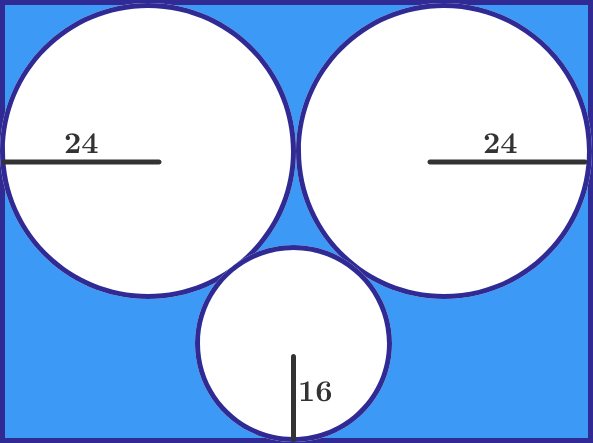# Circles in rectangleIn the diagram above, each circle is in contact two other circles and at least one side of the rectangle. The radii are perpendicular to the sides of the rectangle as shown. Find the area of the shaded portion in $\text{cm}^2$ to the nearest whole number.

All dimensions are in cm.

Use $\pi \approx 3.14159$.

×